Category archive

DIVIDENDS

Forecasting future dividend distributions to see if there is any catalyst for a price change.

TELUS Corporation (NYSE: TU) currently pays a dividend yield of 4.6% which is above the Telecommunication Services sector median of 4.5%. While this makes the total return potential for TELUS Corp look attractive, investors may change their mind when analyzing the company’s future dividends. In this article, I calculate TELUS Corp’s fair value by forecasting its dividend distributions and discounting them back to today’s value.

Valuation Methodologies Are Not Made Equally

The Dividend Discount Model (DDM) estimates the value of a company’s stock price based on the theory that its worth is equal to the sum of the present value of its future dividend payments to shareholders.

But how do we know if it’s appropriate to use a dividend discount analysis when estimating the fair value of TELUS Corp? Many analysts find it difficult when trying to figure out the correct valuation methodology for a given company or are biased towards one specific approach. This is often a mistake which can negatively impact investment decisions and result in trading losses or missed opportunities. No two companies are the same and every business consists of unique characteristics that may require you to adjust your analysis.

Understanding leverage trends is the first step when determining what valuation analyses are relevant for a given company. When a company’s leverage doesn’t fluctuate or is expected to remain stable over time, then an equity valuation model (e.g. equity DCF, DDM) will be the most appropriate valuation technique. The reason for this is because when leverage is stable, interest expense on debt can typically be projected with much more reliability.

How do we check if a company’s leverage has been fluctuating or is expected to do so? This isn’t always straightforward but checking recent debt ratio trends can be a good indicator. TELUS Corp’s debt to equity ratio has been relatively stable over last few years ranging from 147.1% to 166.5%. This suggests that an equity valuation model is a suitable technique when valuing the company’s shares. Now does it make sense to use a dividend discount model knowing that an equity valuation technique is an appropriate methodology?

The next step is to figure out if TELUS Corp pays a dividend and if so, is its payout ratio relatively high (typically above 70%)? The table below highlights this information.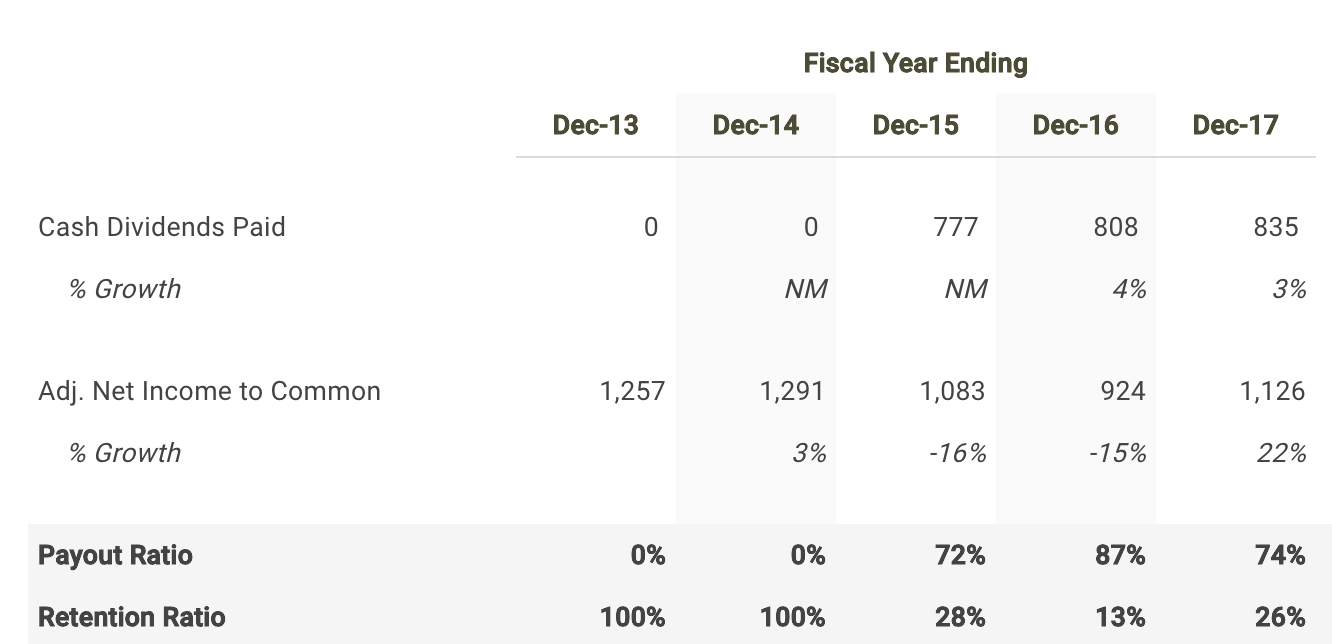Source: TELUS Corp dividend discount model

TELUS Corp distributed a total of \$835 million in cash dividends to shareholders in its most recent fiscal year Dec-17 which represented a payout ratio of 74.1%. It appears that the company meets both criteria. Therefore, it is fitting to use a dividend discount model when determining the fair value of TELUS Corp stock.

Forecasting TELUS Corp’s Dividends

The first step in building a dividend discount model is to forecast net income since forecasting dividends directly can be difficult. So let’s create a net income forecast for the next five years and use that as the basis for projecting future dividends.

As of June 1, Wall Street analysts are projecting a mediocre growth rate in the company’s bottom-line over the next five years. Net income is expected to reach \$1,653 million by fiscal year 2022.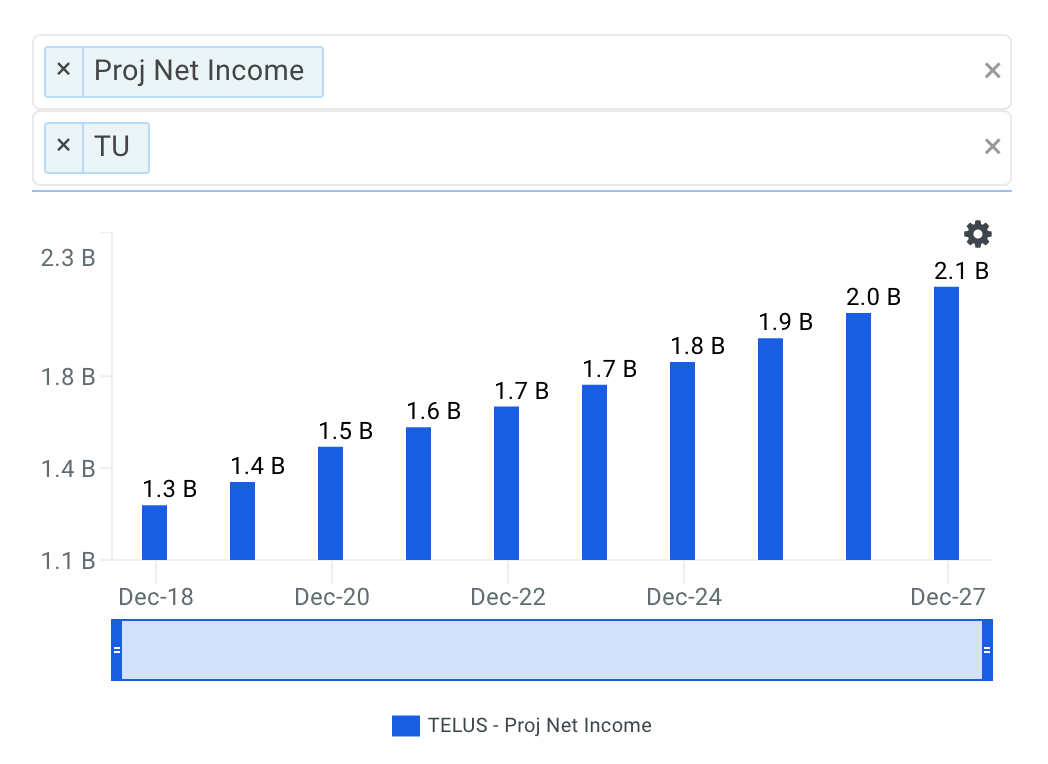Source: TELUS Corp Projected Net Income Growth

I use the net income projections above to serve as the basis for my dividend forecast. The next step is to forecast the payout ratio where I selected 72.0% for the next fiscal year which is in line with historical levels.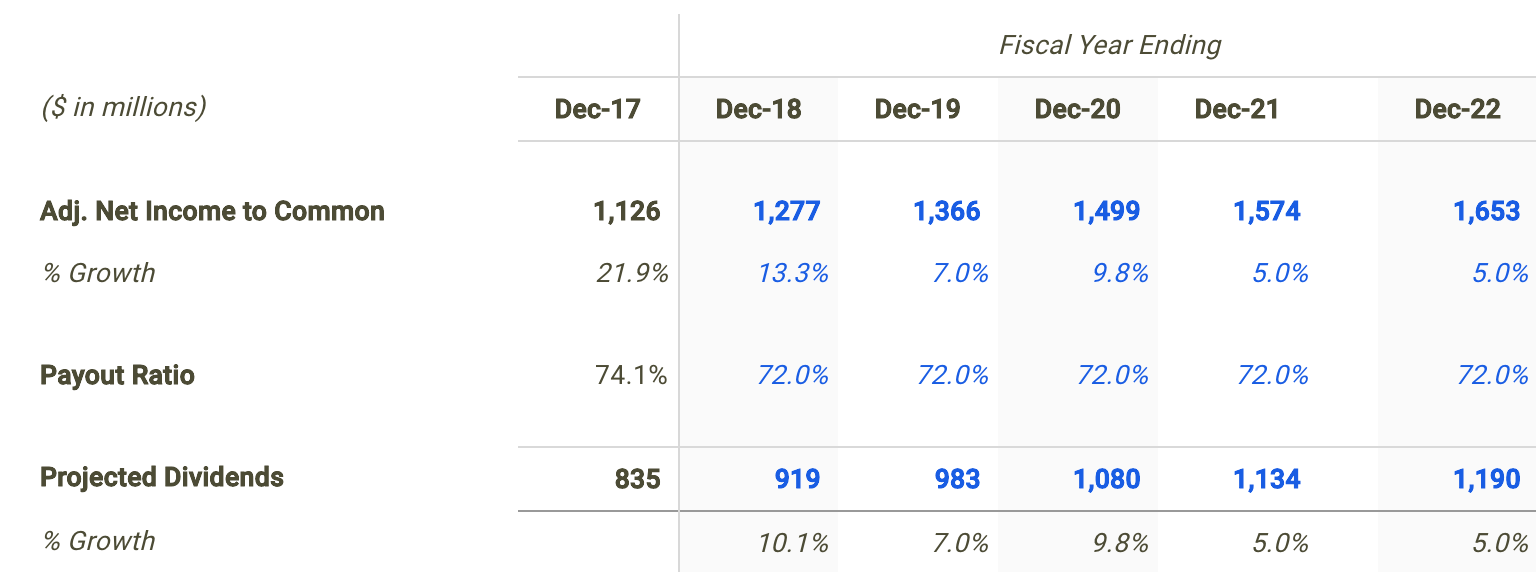Source: TELUS Corp dividend discount model

Calculating TELUS Corp’s Fair Value

The last step is to select a discount rate to calculate the present value of the forecasted dividends. I used finbox.io’s Weighted Average Cost of Capital (WACC) model to help arrive at an estimate for the company’s cost of equity.

I determined a reasonable discount rate for TELUS Corp to be 9.4% at the midpoint. An updated cost of capital analysis using real-time data can be found at finbox.io’s TELUS Corp WACC model page.The assumptions used in the dividend discount model calculate a fair value per share for TELUS Corp of \$35.06, -0.2% below its current stock price of \$35.11. As a result, investors may conclude that they want to hold off on purchasing shares until the stock develops a wider margin of safety.

Conclusion: Dividends Support Stock Price

Discovering the fair value of a company can sometimes be difficult. However, determining an appropriate valuation methodology should not be. Knowing when and when not to use the dividend discount model will help in your investment decision making process.

However, it’s important to understand that a dividend discount model will inherently undervalue a stock. This is typically the result of the payout ratio assumption being less than 100% implying some cash leakage. Meaning the approach does not capture value that would otherwise build up as cash on the balance sheet. In practice, this excess retained cash is usually paid out to shareholders as special dividends or to make up for cash shortfalls for future dividends during economic downturns.

Understanding that this approach calculates a conservative fair value estimate may be a promising sign for investors looking to purchase shares or add to an existing position. Since TU looks to be trading near its intrinsic value based on the analysis above, this could actually represent a ‘downside’ case meaning an ‘upside’ case could have a much larger margin of safety.

Note that there are a number of fundamental factors I have not considered in this analysis. I recommend that you continue your research on TELUS Corp to gain a better understanding of its future prospects.

As of this writing, I did not hold a position in any of the aforementioned securities and this is not a buy or sell recommendation on any security mentioned.

Mercury General Corporation (NYSE: MCY) is currently paying investors an above average dividend yield of 5.3% while the Financials sector median stands at 2.2%. Even though this makes Mercury General look attractive relative to its peers, it’s useful to understand the company’s future dividend potential. Will Mercury General’s bottom line be the main catalyst driving future growth or maybe its payout ratio? Understanding these components and how they impact value may change your mind on the company’s future prospects.

Is A Dividend Analysis Appropriate?

Dividend Discount Model (DDM) is a way of valuing a company based on the theory that a stock is worth the discounted sum of all of its future dividend payments.

However, before walking through my dividend analysis for Mercury General, it’s first helpful to determine if this is actually an appropriate technique to be used when estimating its fair value. Many analysts are often biased towards one specific valuation approach which is typically a mistake that can negatively impact investment decisions and result in trading losses or missed opportunities. Every company has unique characteristics that may require you to adjust your analysis.

Understanding leverage trends is the first step when determining what valuation analyses are relevant for a given company. When a company’s leverage doesn’t fluctuate or is expected to remain stable over time, then an equity valuation model (e.g. equity DCF, DDM) will be the most appropriate valuation technique. The reason for this is because when leverage is stable, interest expense on debt can typically be projected with much more reliability.

How do we check if a company’s leverage has been fluctuating or is expected to do so? This isn’t always straightforward but checking recent debt ratio trends can be a good indicator. Mercury General’s debt to equity ratio has stayed relatively stable over last few years hitting a low of 15.9% in December 2015 and a high of 22.1% in March 2018. This suggests that an equity valuation model is a suitable technique when valuing the company’s shares. Now does it make sense to use a dividend discount model knowing that an equity valuation technique is an appropriate methodology?

We now must determine if Mercury General pays a dividend and if so, is its payout ratio relatively high (typically above 70%)? This helps figure out if the company distributes the majority of its profits to shareholders in the form of dividends.Source: Mercury General dividend discount model

Mercury General paid out a total of \$138 million in cash dividends to shareholders in its most recent fiscal year Dec-17 representing a payout ratio of 95.2%. Therefore, it is fitting to use a dividend discount model when calculating the intrinsic value of Mercury General stock.

How To Project Mercury General’s Dividends

Since forecasting dividends directly can be difficult, the first step in building a dividend discount model is to project net income. So let’s create a net income forecast for the next five years and use that as the basis for our future dividends.

Applying consensus estimates, Wall Street is projecting a healthy growth rate in the company’s bottom-line over the next five years. Net income is expected to grow at an average annual rate of 19% bringing net income to \$344 million by fiscal year 2022.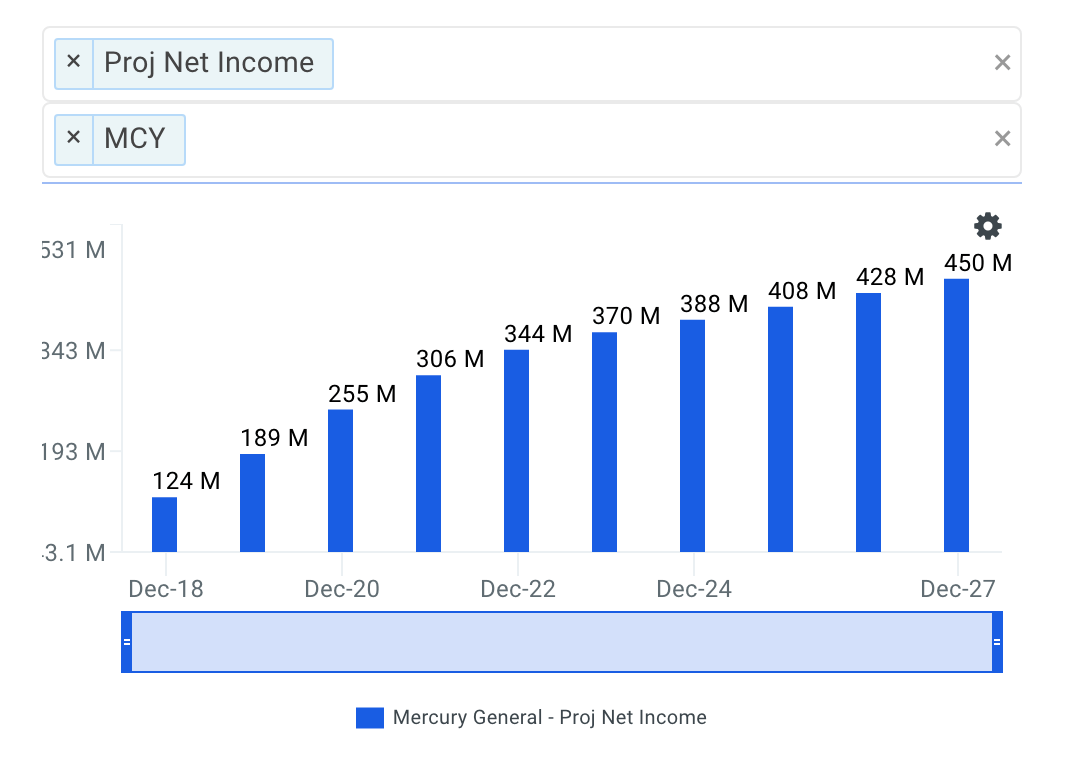Source: Mercury General Projected Net Income Growth

I apply the net profit estimates above to drive my dividend forecast. The next step is to project the company’s payout ratio. In my estimates shown in the table below, I select a 90.0% payout ratio in 2018 and hold it steady there throughout the remainder of my projection period.Source: Mercury General dividend discount model

Discounting Mercury General’s Future Dividends

The final step is to present value the forecasted dividend distributions using a discount rate. I used finbox.io’s Weighted Average Cost of Capital (WACC) model to help arrive at an estimate for the company’s cost of equity.

I concluded a reasonable cost of equity for the company to be 9.7% at the midpoint. Although I don’t walk you through the CAPM assumptions that got me there, you can view the calculations using real-time data at finbox.io’s Mercury General WACC model page.In conclusion, my dividend discount model calculates a fair value per share for Mercury General of \$63.57, 34.3% above its current stock price of \$47.34. MCY appears to be an undervalued stock based on the company’s future dividend potential alone. Therefore, now may be a good time to purchase shares or increase your position in the company.

What This Means For Investors

An important component of the dividend discount model that investors should understand is that the technique will inherently undervalue a stock. This is typically the result of the payout ratio assumption being less than 100% implying some cash leakage. Meaning the approach does not capture value that would otherwise build up as cash on the balance sheet. In practice, this excess retained cash is usually paid out to shareholders as special dividends or to make up for cash shortfalls for future dividends during economic downturns.

Understanding that this approach calculates a conservative intrinsic value estimate is a very promising sign for investors searching for an entry point to buy the stock. Shares of Mercury General currently appear to be undervalued based on its future dividend potential alone. This means that MCY may have significantly more upside than what I’ve calculated above.

Although a dividend discount model on its own is not necessarily indicative of a stock’s fair value, it does offer a number of useful insights. This valuation should only be the start of your analysis in relation to your total research. I recommend that you continue your review of Mercury General to gain a better understanding of its underlying fundamentals.

As of this writing, I did not hold a position in any of the aforementioned securities and this is not a buy or sell recommendation on any security mentioned.

TransMontaigne Partners (NYSE: TLP) has distributed 144.6% of its profits in the form of dividends over the last twelve months making the stock a prime candidate to be valued using a dividend discount analysis. Operating as an energy company paying a dividend yield of 8.0%, could the small-cap stock be trading at an attractive valuation? Let’s take a look at the future dividend potential of TransMontaigne to see if there is any catalyst for a price change.

Application Of The Dividend Discount Model

The Dividend Discount Model (DDM) is a dividend-based valuation model that estimates the present value of a stock based on assumptions about its future dividend performance.

But before discussing the assumptions used in my dividend discount model for TransMontaigne, it’s first helpful to determine if this is actually an appropriate technique to be used when estimating its fair value. Many analysts find it difficult when trying to figure out the correct valuation methodology for a given company or are biased towards one specific approach. This is often a mistake which can negatively impact investment decisions and result in trading losses or missed opportunities. No two companies are the same and every business consists of unique characteristics that may require you to adjust your analysis.

Understanding leverage trends is the first step when determining what valuation analyses are relevant for a given company. When a company’s leverage doesn’t fluctuate or is expected to remain stable over time, then an equity valuation model (e.g. equity DCF, DDM) will be the most appropriate valuation technique. The reason for this is because when leverage is stable, interest expense on debt can typically be projected with much more reliability.

How do we check if a company’s leverage has been fluctuating or is expected to do so? This isn’t always straightforward but checking recent debt ratio trends can be a good indicator. TransMontaigne’s debt to equity ratio has been relatively consistent over last few years with a range spanning only 17.4%. This suggests that an equity valuation model is a suitable technique when valuing the company’s shares. Now does it make sense to use a dividend discount model knowing that an equity valuation technique is an appropriate methodology?

The next step is to determine if TransMontaigne pays a dividend and if so, is its payout ratio relatively high (typically above 70%)? The table below provides this information in more detail.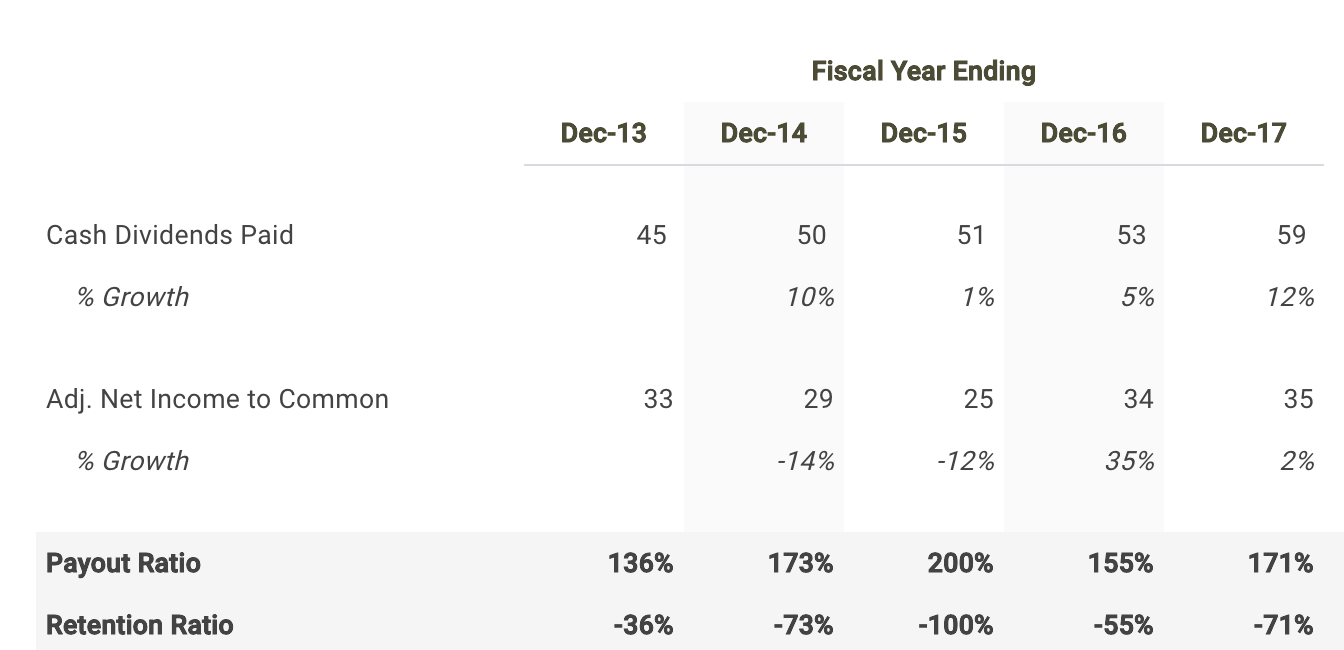Source: TransMontaigne dividend discount model

TransMontaigne distributed a total of \$53 million in cash dividends to shareholders in its most recent fiscal year Dec-17 which represented a payout ratio of 152.8%. It appears that the company meets both criteria. Therefore, it is fitting to apply a dividend discount model when calculating the intrinsic value of TransMontaigne.

Projecting The Future Dividends Of TransMontaigne

The first step in building a dividend discount model is to forecast net income since forecasting dividends directly can be difficult. So let’s create a net income forecast for the next five years and use that as the basis for projecting future dividends.

Finbox.io applies consensus Wall Street estimates for the net income forecast when available. For the next fiscal year 2018, profits are expected to increase 12.2%, then grow 48.4% in 2019 and fall -10.2% in 2020.Source: TransMontaigne Projected Net Income Growth

I use the projections above to serve as the basis for my dividend projections. The next step is to forecast the company’s payout ratio. In my estimates shown in the table below, I select a 90.0% payout ratio in 2018 and hold it steady there throughout the remainder of my projection period.Source: TransMontaigne dividend discount model

Calculating TransMontaigne’s Intrinsic Value

Finally, we can now calculate TransMontaigne’s intrinsic value by present valuing its forecasted dividends. Note that we apply the company’s cost of equity to discount the future dividends since these payments are made to common shareholders or equity owners. I used finbox.io’s Weighted Average Cost of Capital (WACC) model to help arrive at an estimate for the company’s cost of equity.

I determined a reasonable cost of equity for TransMontaigne to be 15.3% at the midpoint. An updated cost of capital analysis using real-time data can be found at finbox.io’s TransMontaigne WACC model page.My dividend forecast and cost of capital assumptions imply a fair value per share for TransMontaigne of \$24.58, -35.3% below its current stock price of \$37.65. Therefore, TLP appears to be an overvalued stock and not necessarily a good entry point opportunity.

Conclusion: Dividends Don’t Support Stock Price

Finding the true value of a company can sometimes be difficult but determining an appropriate valuation methodology should not be. Knowing when and when not to use the dividend discount model will help in your investment decision making process.

But it is important to note that a dividend discount model will inherently undervalue a company’s stock. This is typically the result of the payout ratio assumption being less than 100% implying some cash leakage. In reality, this excess retained cash is usually paid out to shareholders as special dividends or to make up for cash shortfalls for future dividends during economic downturns.

This helps explain, at least partially, why TransMontaigne looks like an overvalued stock based on this approach. Nevertheless, a dividend discount analysis is still a helpful tool in combination with the understanding that it calculates a conservative intrinsic value estimate. Meaning an extremely attractive opportunity would be a stock where (1) a dividend discount model is appropriate and (2) shares looks undervalued based on its future dividends.

While a dividend discount analysis on its own is not necessarily indicative of a stock’s intrinsic value, it does provide helpful insights. I recommend that investors continue their research on TransMontaigne to gain a better understanding of all the factors driving its share price.

As of this writing, I did not hold a position in any of the aforementioned securities and this is not a buy or sell recommendation on any security mentioned.

LTC Properties Inc (NYSE: LTC) currently pays a dividend yield of 5.8% which is above the Real Estate sector median of 4.6%. While this makes the total return potential for LTC look attractive, investors may change their mind when analyzing the company’s future dividends. In this article, I calculate LTC’s fair value by forecasting its dividend distributions and discounting them back to today’s value.

Valuation Methodologies Are Not Made Equally

The Dividend Discount Model (DDM) estimates the value of a company’s stock price based on the theory that its worth is equal to the sum of the present value of its future dividend payments to shareholders.

But how do we know if it’s appropriate to use a dividend discount analysis when estimating the fair value of LTC? Many analysts find it difficult when trying to figure out the correct valuation methodology for a given company or are biased towards one specific approach. This is often a mistake which can negatively impact investment decisions and result in trading losses or missed opportunities. No two companies are the same and every business consists of unique characteristics that may require you to adjust your analysis.

Understanding leverage trends is the first step when determining what valuation analyses are relevant for a given company. When a company’s leverage doesn’t fluctuate or is expected to remain stable over time, then an equity valuation model (e.g. equity DCF, DDM) will be the most appropriate valuation technique. The reason for this is because when leverage is stable, interest expense on debt can typically be projected with much more reliability.

How do we check if a company’s leverage has been fluctuating or is expected to do so? This isn’t always straightforward but checking recent debt ratio trends can be a good indicator. LTC’s debt to equity ratio has been relatively stable over last few years ranging from 82.3% to 88.0%. This suggests that an equity valuation model is a suitable technique when valuing the company’s shares. Now does it make sense to use a dividend discount model knowing that an equity valuation technique is an appropriate methodology?

The next step is to figure out if LTC pays a dividend and if so, is its payout ratio relatively high (typically above 70%)? The table below highlights this information.Source: LTC dividend discount model

LTC distributed a total of \$90 million in cash dividends to shareholders in its most recent fiscal year Dec-17 which represented a payout ratio of 103.7%. It appears that the company meets both criteria. Therefore, it is fitting to use a dividend discount model when determining the fair value of LTC stock.

Forecasting LTC’s Dividends

The first step in building a dividend discount model is to forecast net income since forecasting dividends directly can be difficult. So let’s create a net income forecast for the next five years and use that as the basis for projecting future dividends.

As of May 24, Wall Street analysts are projecting a mediocre growth rate in the company’s bottom-line over the next five years. Net income is expected to reach \$102 million by fiscal year 2022.Source: LTC Projected Net Income Growth

I use the net income projections above to serve as the basis for my dividend forecast. The next step is to forecast the payout ratio where I selected 90.0% for the next fiscal year which is in line with historical levels.Source: LTC dividend discount model

Calculating LTC’s Fair Value

The last step is to select a discount rate to calculate the present value of the forecasted dividends. I used finbox.io’s Weighted Average Cost of Capital (WACC) model to help arrive at an estimate for the company’s cost of equity.

I determined a reasonable discount rate for LTC to be 9.7% at the midpoint. An updated cost of capital analysis using real-time data can be found at finbox.io’s LTC WACC model page.The assumptions used in the dividend discount model calculate a fair value per share for LTC of \$34.28, -13.4% below its current stock price of \$39.52. Therefore, LTC appears to be an overvalued stock and not necessarily a good entry point opportunity.

Conclusion: Dividends Don’t Support Stock Price

Discovering the fair value of a company can sometimes be difficult. However, determining an appropriate valuation methodology should not be. Knowing when and when not to use the dividend discount model will help in your investment decision making process.

However, it’s important to understand that a dividend discount model will inherently undervalue a stock. This is typically the result of the payout ratio assumption being less than 100% implying some cash leakage. Meaning the approach does not capture value that would otherwise build up as cash on the balance sheet. In practice, this excess retained cash is usually paid out to shareholders as special dividends or to make up for cash shortfalls for future dividends during economic downturns.

This helps explain, at least partially, why LTC looks like an overvalued stock based on this approach. Nevertheless, a dividend discount analysis is still a helpful tool in combination with the understanding that it calculates a conservative intrinsic value estimate. Meaning an extremely attractive opportunity would be a stock where (1) a dividend discount model is appropriate and (2) shares looks undervalued based on its future dividends.

Note that there are a number of fundamental factors I have not considered in this analysis. I recommend that you continue your research on LTC to gain a better understanding of its future prospects.

As of this writing, I did not hold a position in any of the aforementioned securities and this is not a buy or sell recommendation on any security mentioned.

Western Gas Equity Partners (NYSE: WGP) has distributed 224.4% of its profits in the form of dividends over the last twelve months making the stock a prime candidate to be valued using a dividend discount analysis. Operating as an energy company paying a dividend yield of 6.3%, could the mid-cap stock be trading at an attractive valuation? Let’s take a look at the future dividend potential of WGP to see if there is any catalyst for a price change.

Application Of The Dividend Discount Model

The Dividend Discount Model (DDM) is a dividend-based valuation model that estimates the present value of a stock based on assumptions about its future dividend performance.

But before discussing the assumptions used in my dividend discount model for WGP, it’s first helpful to determine if this is actually an appropriate technique to be used when estimating its fair value. Many analysts find it difficult when trying to figure out the correct valuation methodology for a given company or are biased towards one specific approach. This is often a mistake which can negatively impact investment decisions and result in trading losses or missed opportunities. No two companies are the same and every business consists of unique characteristics that may require you to adjust your analysis.

Understanding leverage trends is the first step when determining what valuation analyses are relevant for a given company. When a company’s leverage doesn’t fluctuate or is expected to remain stable over time, then an equity valuation model (e.g. equity DCF, DDM) will be the most appropriate valuation technique. The reason for this is because when leverage is stable, interest expense on debt can typically be projected with much more reliability.

How do we check if a company’s leverage has been fluctuating or is expected to do so? This isn’t always straightforward but checking recent debt ratio trends can be a good indicator. WGP’s debt to equity ratio has been relatively consistent over last few years with a range spanning only 15.1%. This suggests that an equity valuation model is a suitable technique when valuing the company’s shares. Now does it make sense to use a dividend discount model knowing that an equity valuation technique is an appropriate methodology?

The next step is to determine if WGP pays a dividend and if so, is its payout ratio relatively high (typically above 70%)? The table below provides this information in more detail.Source: WGP dividend discount model

WGP distributed a total of \$811 million in cash dividends to shareholders in its most recent fiscal year Dec-17 which represented a payout ratio of 215.4%. It appears that the company meets both criteria. Therefore, it is fitting to apply a dividend discount model when calculating the intrinsic value of WGP.

Projecting The Future Dividends Of WGP

The first step in building a dividend discount model is to forecast net income since forecasting dividends directly can be difficult. So let’s create a net income forecast for the next five years and use that as the basis for projecting future dividends.

Finbox.io applies consensus Wall Street estimates for the net income forecast when available. For the next fiscal year 2018, profits are expected to increase 13.4%, then grow 10.9% in 2019 and rise 15.8% in 2020.Source: WGP Projected Net Income Growth

I use the projections above to serve as the basis for my dividend projections. The next step is to forecast the company’s payout ratio. In my estimates shown in the table below, I select a 90.0% payout ratio in 2018 and hold it steady there throughout the remainder of my projection period.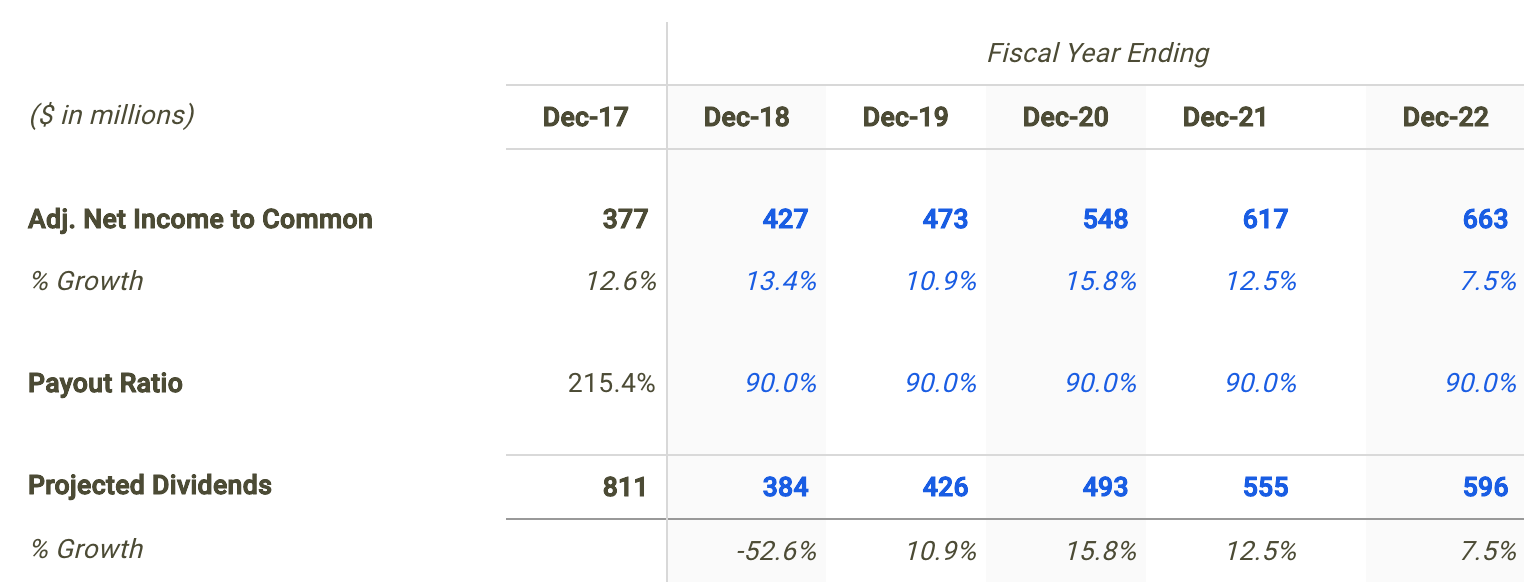Source: WGP dividend discount model

Calculating WGP’s Intrinsic Value

Finally, we can now calculate WGP’s intrinsic value by present valuing its forecasted dividends. Note that we apply the company’s cost of equity to discount the future dividends since these payments are made to common shareholders or equity owners. I used finbox.io’s Weighted Average Cost of Capital (WACC) model to help arrive at an estimate for the company’s cost of equity.

I determined a reasonable cost of equity for WGP to be 11.9% at the midpoint. An updated cost of capital analysis using real-time data can be found at finbox.io’s WGP WACC model page.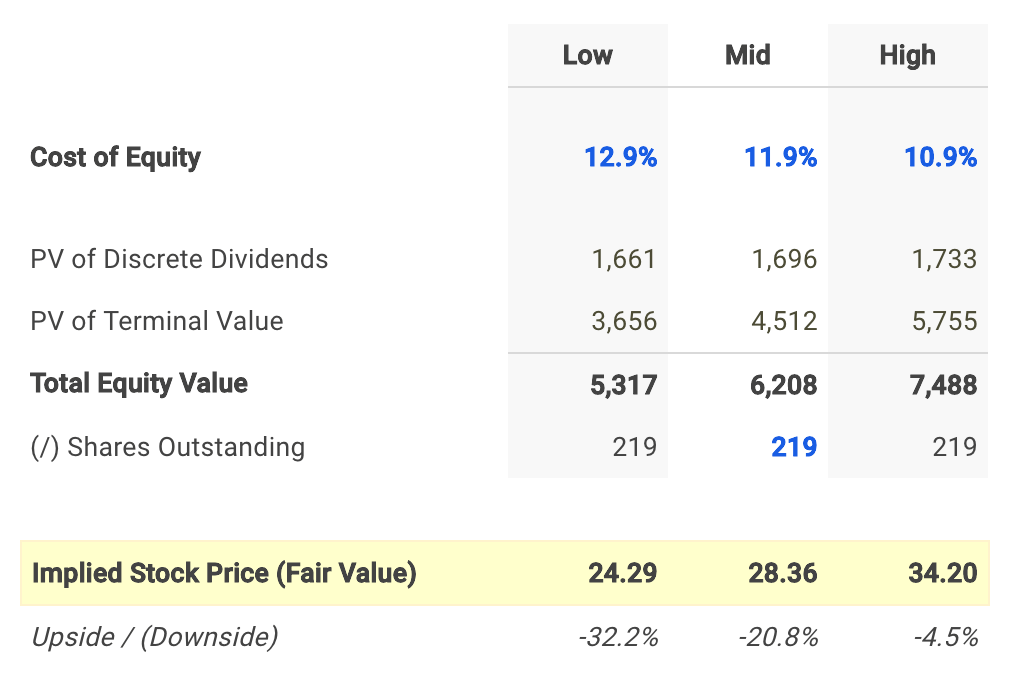My dividend forecast and cost of capital assumptions imply a fair value per share for WGP of \$28.36, -20.8% below its current stock price of \$35.69. Therefore, WGP appears to be an overvalued stock and not necessarily a good entry point opportunity.

Conclusion: Dividends Don’t Support Stock Price

Finding the true value of a company can sometimes be difficult but determining an appropriate valuation methodology should not be. Knowing when and when not to use the dividend discount model will help in your investment decision making process.

But it is important to note that a dividend discount model will inherently undervalue a company’s stock. This is typically the result of the payout ratio assumption being less than 100% implying some cash leakage. In reality, this excess retained cash is usually paid out to shareholders as special dividends or to make up for cash shortfalls for future dividends during economic downturns.

This helps explain, at least partially, why WGP looks like an overvalued stock based on this approach. Nevertheless, a dividend discount analysis is still a helpful tool in combination with the understanding that it calculates a conservative intrinsic value estimate. Meaning an extremely attractive opportunity would be a stock where (1) a dividend discount model is appropriate and (2) shares looks undervalued based on its future dividends.

While a dividend discount analysis on its own is not necessarily indicative of a stock’s intrinsic value, it does provide helpful insights. I recommend that investors continue their research on WGP to gain a better understanding of all the factors driving its share price.

As of this writing, I did not hold a position in any of the aforementioned securities and this is not a buy or sell recommendation on any security mentioned.

Hawaiian Electric Industries, Inc (NYSE: HE) is currently paying investors an above average dividend yield of 3.8% while the Utilities sector median stands at 3.5%. Even though this makes Hawaiian Electric look attractive relative to its peers, it’s useful to understand the company’s future dividend potential. Will Hawaiian Electric’s bottom line be the main catalyst driving future growth or maybe its payout ratio? Understanding these components and how they impact value may change your mind on the company’s future prospects.

Is A Dividend Analysis Appropriate?

Dividend Discount Model (DDM) is a way of valuing a company based on the theory that a stock is worth the discounted sum of all of its future dividend payments.

However, before walking through my dividend analysis for Hawaiian Electric, it’s first helpful to determine if this is actually an appropriate technique to be used when estimating its fair value. Many analysts are often biased towards one specific valuation approach which is typically a mistake that can negatively impact investment decisions and result in trading losses or missed opportunities. Every company has unique characteristics that may require you to adjust your analysis.

Understanding leverage trends is the first step when determining what valuation analyses are relevant for a given company. When a company’s leverage doesn’t fluctuate or is expected to remain stable over time, then an equity valuation model (e.g. equity DCF, DDM) will be the most appropriate valuation technique. The reason for this is because when leverage is stable, interest expense on debt can typically be projected with much more reliability.

How do we check if a company’s leverage has been fluctuating or is expected to do so? This isn’t always straightforward but checking recent debt ratio trends can be a good indicator. Hawaiian Electric’s debt to equity ratio has stayed relatively stable over last few years hitting a low of 87.7% in December 2016 and a high of 104.3% in December 2015. This suggests that an equity valuation model is a suitable technique when valuing the company’s shares. Now does it make sense to use a dividend discount model knowing that an equity valuation technique is an appropriate methodology?

We now must determine if Hawaiian Electric pays a dividend and if so, is its payout ratio relatively high (typically above 70%)? This helps figure out if the company distributes the majority of its profits to shareholders in the form of dividends.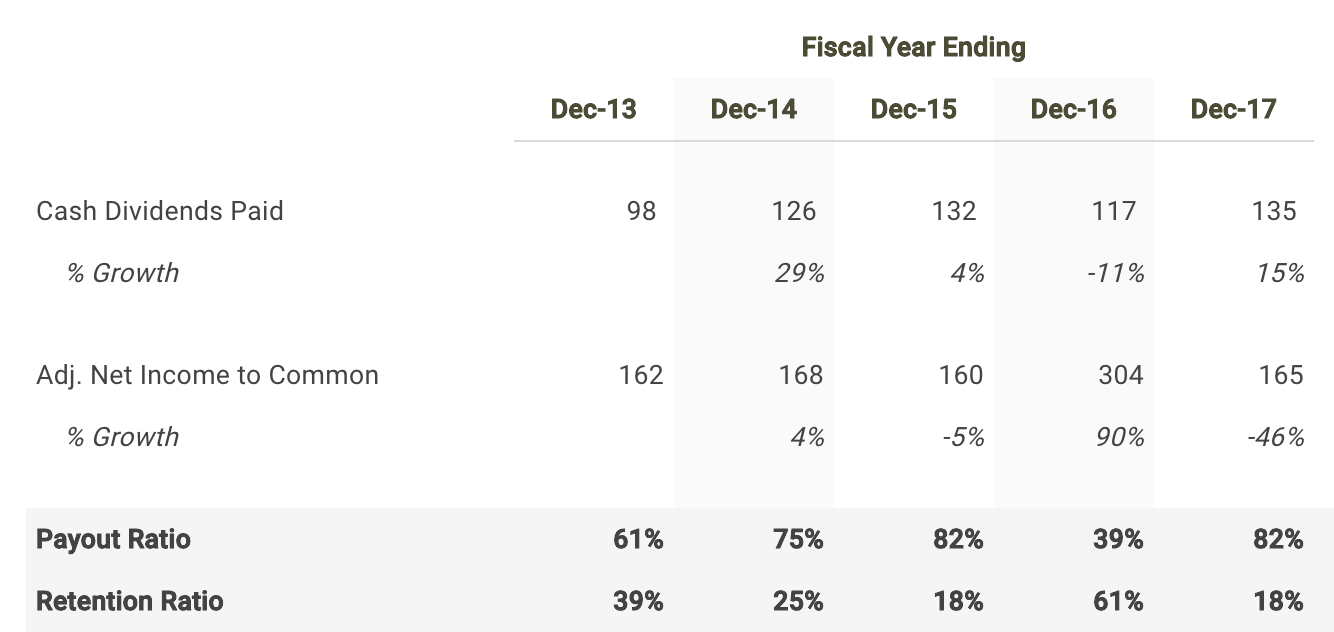Source: Hawaiian Electric dividend discount model

Hawaiian Electric paid out a total of \$135 million in cash dividends to shareholders in its most recent fiscal year Dec-17 representing a payout ratio of 81.6%. Therefore, it is fitting to use a dividend discount model when calculating the intrinsic value of Hawaiian Electric stock.

How To Project Hawaiian Electric’s Dividends

Since forecasting dividends directly can be difficult, the first step in building a dividend discount model is to project net income. So let’s create a net income forecast for the next five years and use that as the basis for our future dividends.

Applying consensus estimates, Wall Street is projecting a mediocre growth rate in the company’s bottom-line over the next five years. Net income is expected to grow at an average annual rate of 10% bringing net income to \$260 million by fiscal year 2022.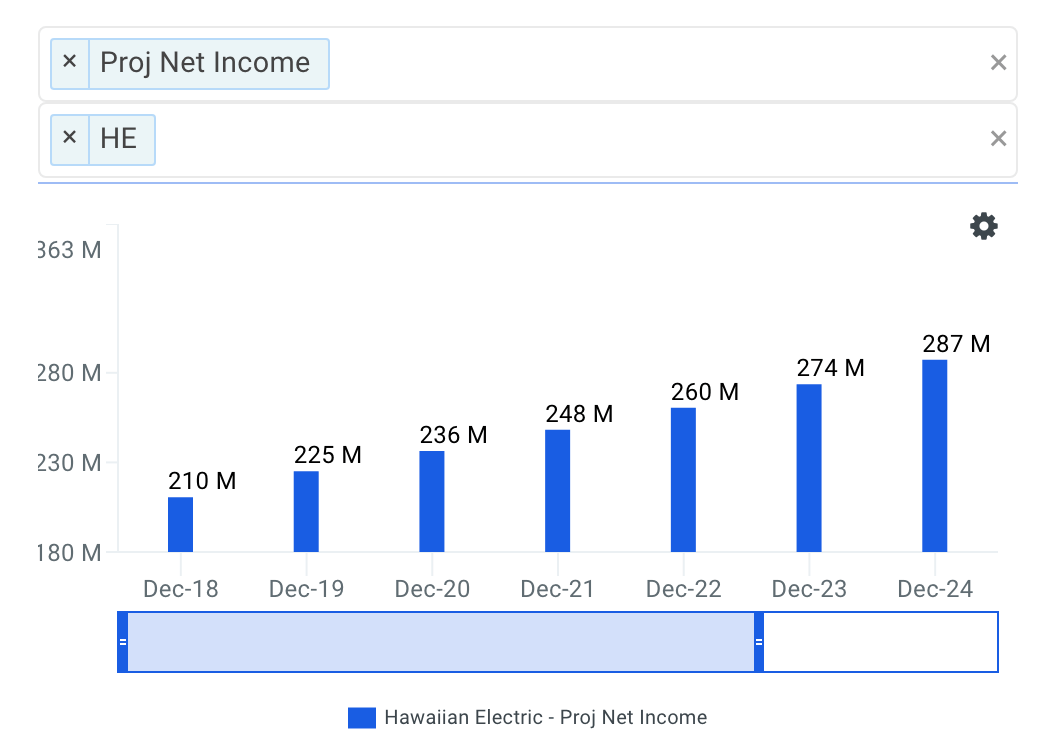Source: Hawaiian Electric Projected Net Income Growth

I apply the net profit estimates above to drive my dividend forecast. The next step is to project the company’s payout ratio. In my estimates shown in the table below, I select a 75.0% payout ratio in 2018 and hold it steady there throughout the remainder of my projection period.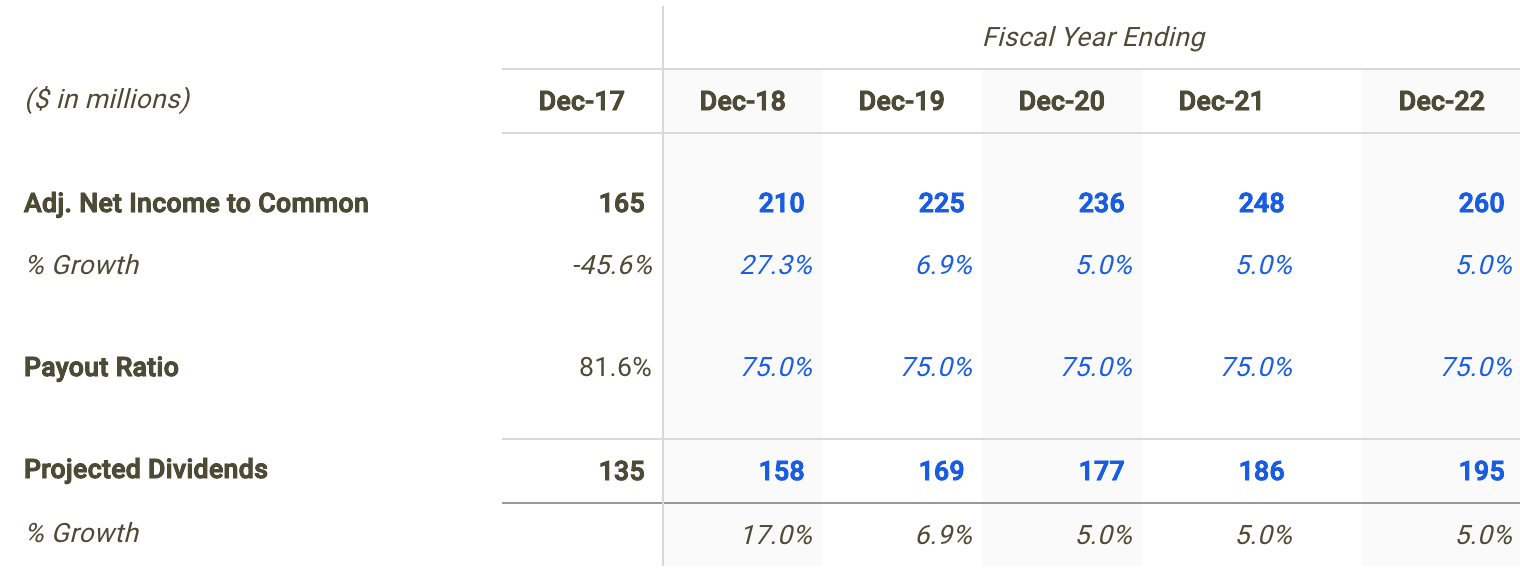Source: Hawaiian Electric dividend discount model

Discounting Hawaiian Electric’s Future Dividends

The final step is to present value the forecasted dividend distributions using a discount rate. I used finbox.io’s Weighted Average Cost of Capital (WACC) model to help arrive at an estimate for the company’s cost of equity.

I concluded a reasonable cost of equity for the company to be 9.6% at the midpoint. Although I don’t walk you through the CAPM assumptions that got me there, you can view the calculations using real-time data at finbox.io’s Hawaiian Electric WACC model page.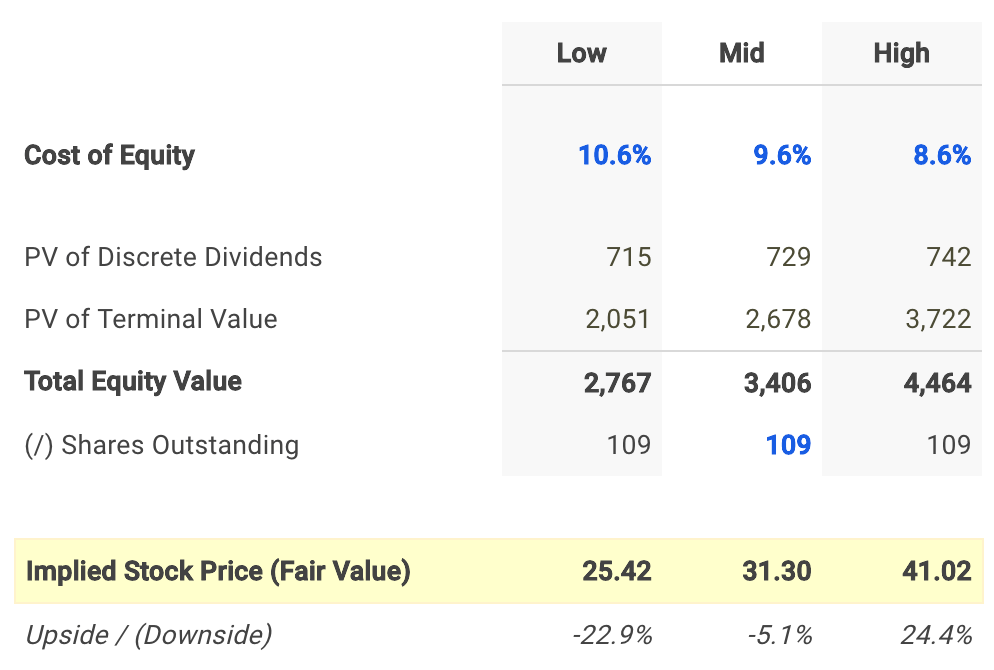In conclusion, my dividend discount model calculates a fair value per share for Hawaiian Electric of \$31.30, -6.4% below its current stock price of \$33.45. As a result, investors may conclude that they want to hold off on purchasing shares until the stock develops a wider margin of safety.

What This Means For Investors

An important component of the dividend discount model that investors should understand is that the technique will inherently undervalue a stock. This is typically the result of the payout ratio assumption being less than 100% implying some cash leakage. Meaning the approach does not capture value that would otherwise build up as cash on the balance sheet. In practice, this excess retained cash is usually paid out to shareholders as special dividends or to make up for cash shortfalls for future dividends during economic downturns.

Understanding that this approach calculates a conservative fair value estimate may be a promising sign for investors looking to purchase shares or add to an existing position. Since HE looks to be trading near its intrinsic value based on the analysis above, this could actually represent a ‘downside’ case meaning an ‘upside’ case could have a much larger margin of safety.

Although a dividend discount model on its own is not necessarily indicative of a stock’s fair value, it does offer a number of useful insights. This valuation should only be the start of your analysis in relation to your total research. I recommend that you continue your review of Hawaiian Electric to gain a better understanding of its underlying fundamentals.

As of this writing, I did not hold a position in any of the aforementioned securities and this is not a buy or sell recommendation on any security mentioned.

1 2 3 6
Go to Top